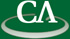## Linear Hensel lifting for Zp[x,y] for n factors with cubic cost

### Garrett Paluck, CECM, Simon Fraser Univeristy

Friday March 11th, 12:30pm, online.

Abstract:

We present an algorithm for performing linear Hensel lifting on bivariate polynomials over the finite field Zp for some prime p. Our algorithm lifts n monic, univariate polynomials to recover the factors of a polynomial A(x,y) in Zp[x,y] which is monic in x, and bounded by degrees dx = deg(A,x) and dy = deg(A,y). Our algorithm improves upon Bernardin's algorithm and reduces the number of arithmetic operations in Zp from O(n dx^2 dy^2) to O(dx^2 dy + dx dy^2) and the space complexity from O(n dx dy) to O(log_2(n) dx dy) elements of Zp for p >= dx.